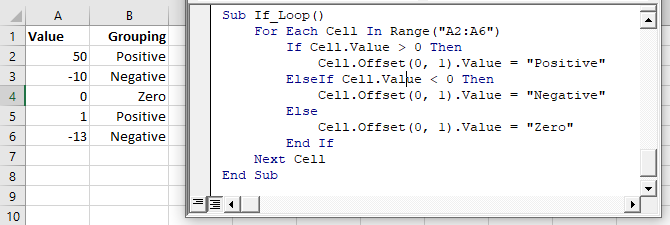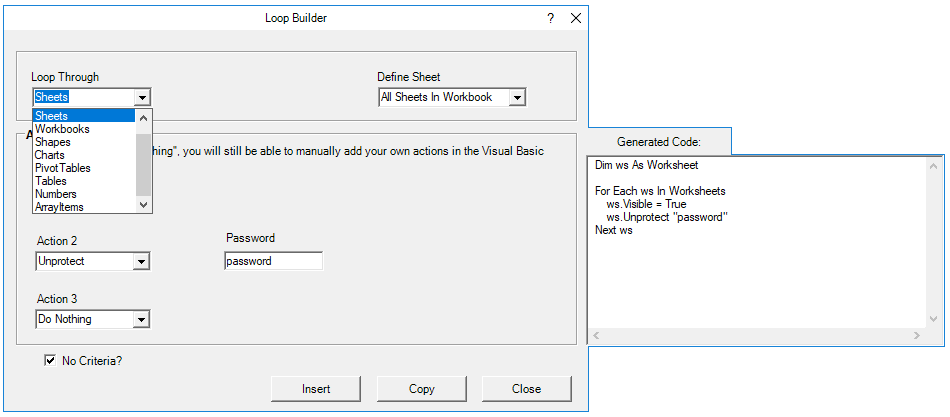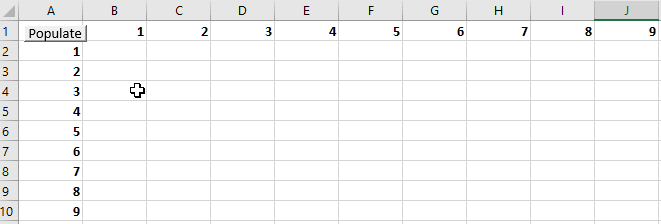# Excel VBA Loops – For Each, For Next, Do While, Nested & More

To work effectively in VBA, you must understand Loops.

Loops allow you to repeat a code block a set number of times or repeat a code block on a each object in a set of objects.

First we will show you a few examples to show you what loops are capable of. Then we will teach you everything about loops.

## VBA Loop Quick Examples

### For Each Loops

For Each Loops loop through every object in a collection, such as every worksheet in workbook or every cell in a range.

#### Loop Through all Worksheets in Workbook

This code will loop through all worksheets in the workbook, unhiding each sheet:

``````Sub LoopThroughSheets()
Dim ws As Worksheet

For Each ws In Worksheets
ws.Visible = True
Next

End Sub``````

#### Loop Through All Cells in Range

This code will loop through a range of cells, testing if the cell value is negative, positive, or zero:

``````Sub If_Loop()
Dim Cell as Range

For Each Cell In Range("A2:A6")
If Cell.Value > 0 Then
Cell.Offset(0, 1).Value = "Positive"
ElseIf Cell.Value < 0 Then
Cell.Offset(0, 1).Value = "Negative"
Else
Cell.Offset(0, 1).Value = "Zero"
End If
Next Cell

End Sub``````### For Next Loops

Another type of “For” Loop is the For Next Loop.  The For Next Loop allows you to loop through integers.

This code will loop through integers 1 through 10, displaying each with a message box:

``````Sub ForLoop()
Dim i As Integer
For i = 1 To 10
MsgBox i
Next i
End Sub``````

### Do While Loops

Do While Loops will loop while a condition is met. This code will also loop through integers 1 through 10, displaying each with a message box.

``````Sub DoWhileLoop()
Dim n As Integer
n = 1
Do While n < 11
MsgBox n
n = n + 1
Loop
End Sub``````

### Do Until Loops

Conversely, Do Until Loops will loop until a condition is met. This code does the same thing as the previous two examples.

``````Sub DoUntilLoop()
Dim n As Integer
n = 1
Do Until n >= 10
MsgBox n
n = n + 1
Loop
End Sub``````

We will discuss this below, but you need to be extremely careful when creating Do While or Do Until loops so that you don’t create a never ending loop.

## VBA Loop BuilderThis is a screenshot of the “Loop Builder” from our Premium VBA Add-in: AutoMacro. The Loop Builder allows you to quickly and easily build loops to loop through different objects, or numbers. You can perform actions on each object and/or select only objects that meet certain criteria.

The add-in also contains many other code builders, an extensive VBA code library, and an assortment of coding tools. It’s a must have for any VBA developer.

Now we will cover the different types of loops in depth.

## VBA For Next Loop

### For Loop Syntax

The For Next Loop allows you to repeat a block of code a specified number of times. The syntax is:

``````[Dim Counter as Integer]

For Counter = Start to End [Step Value]
[Do Something]
Next [Counter]``````

Where the items in brackets are optional.

• [Dim Counter as Long] – Declares the counter variable. Required if Option Explicit is declared at the top of your module.
• Counter – An integer variable used to count
• Start – The start value (Ex. 1)
• End – The end value (Ex. 10)
• [Step Value] – Allows you to count every n integers instead of every 1 integer. You can also go in reverse with a negative value (ex. Step -1)
• [Do Something] – The code that will repeat
• Next [Counter] – Closing statement to the For Next Loop. You can include the Counter or not. However, I strongly recommend including the counter as it makes your code easier to read.

If that’s confusing, don’t worry. We will review some examples:

#### Count to 10

This code will count to 10 using a For-Next Loop:

``````Sub ForEach_CountTo10()

Dim n As Integer
For n = 1 To 10
MsgBox n
Next n

End Sub``````

### For Loop Step

#### Count to 10 – Only Even Numbers

This code will count to 10 only counting even numbers:

``````Sub ForEach_CountTo10_Even()

Dim n As Integer
For n = 2 To 10 Step 2
MsgBox n
Next n

End Sub``````

Notice we added “Step 2”. This tells the For Loop to “step” through the counter by 2.  We can also use a negative step value to step in reverse:

VBA Programming | Code Generator does work for you!

### For Loop Step – Inverse

#### Countdown from 10

This code will countdown from 10:

``````Sub ForEach_Countdown_Inverse()

Dim n As Integer
For n = 10 To 1 Step -1
MsgBox n
Next n
MsgBox "Lift Off"

End Sub``````

#### Delete Rows if Cell is Blank

I’ve most frequently used a negative step For-Loop to loop through ranges of cells, deleting rows that meet certain criteria.  If you loop from the top rows to the bottom rows, as you delete rows you will mess up your counter.

This example will delete rows with blank cells (starting from the bottom row):

``````Sub ForEach_DeleteRows_BlankCells()

Dim n As Integer
For n = 10 To 1 Step -1
If Range("a" & n).Value = "" Then
Range("a" & n).EntireRow.Delete
End If
Next n

End Sub``````

### Nested For Loop

You can “nest” one For Loop inside another For Loop. We will use Nested For Loops to create a multiplication table:

``````Sub Nested_ForEach_MultiplicationTable()

Dim row As Integer, col As Integer

For row = 1 To 9
For col = 1 To 9
Cells(row + 1, col + 1).Value = row * col
Next col
Next row

End Sub``````### Exit For

The Exit For statement allows you to exit a For Next loop immediately.

You would usually use Exit For along with an If Statement, exiting the For Next Loop if a certain condition is met.

For example, you might use a For Loop to find a cell. Once that cell is found, you can exit the loop to speed up your code.

This code will loop through rows 1 to 1000, looking for “error” in column A. If it’s found, the code will select the cell, alert you to the found error, and exit the loop:

``````Sub ExitFor_Loop()

Dim i As Integer

For i = 1 To 1000
If Range("A" & i).Value = "error" Then
Range("A" & i).Select
MsgBox "Error Found"
Exit For
End If
Next i

End Sub``````

Important: In the case of Nested For Loops, Exit For only exits the current For Loop, not all active Loops.

### Continue For

VBA does not have the “Continue” command that’s found in Visual Basic. Instead, you will need to use “Exit”.

## VBA For Each Loop

The VBA For Each Loop will loop through all objects in a collection:

• All cells in a range
• All worksheets in a workbook
• All shapes in a worksheet
• All open workbooks

You can also use Nested For Each Loops to:

• All cells in a range on all worksheets
• All shapes on all worksheets
• All sheets in all open workbooks
• and so on…

The syntax is:

``````For Each Object in Collection
[Do Something]
Next [Object]``````

Where:

• Object – Variable representing a Range, Worksheet, Workbook, Shape, etc. (ex. rng)
• Collection – Collection of objects (ex. Range(“a1:a10”)
• [Do Something] – Code block to run on each object
• Next [Object] – Closing statement. [Object] is optional, however strongly recommended.

### For Each Cell in Range

This code will loop through each cell in a range:

``````Sub ForEachCell_inRange()

Dim cell As Range

For Each cell In Range("a1:a10")
cell.Value = cell.Offset(0,1).Value
Next cell

End Sub``````

### For Each Worksheet in Workbook

This code will loop through all worksheets in a workbook, unprotecting each sheet:

``````Sub ForEachSheet_inWorkbook()

Dim ws As Worksheet

For Each ws In Worksheets
Next ws

End Sub``````

### For Each Open Workbook

This code will save and close all open workbooks:

``````Sub ForEachWB_inWorkbooks()

Dim wb As Workbook

For Each wb In Workbooks
wb.Close SaveChanges:=True
Next wb

End Sub``````

### For Each Shape in Worksheet

This code will delete all shapes in the active sheet.

``````Sub ForEachShape()

Dim shp As Shape

For Each shp In ActiveSheet.Shapes
shp.Delete
Next shp

End Sub
``````

### For Each Shape in Each Worksheet in Workbook

You can also nest For Each Loops. Here we will loop through all shapes in all worksheets in the active workbook:

``````Sub ForEachShape_inAllWorksheets()

Dim shp As Shape, ws As Worksheet

For Each ws In Worksheets
For Each shp In ws.Shapes
shp.Delete
Next shp
Next ws

End Sub``````

### For Each – IF Loop

As we’ve mentioned before, you can use an If statement within a loop, performing actions only if certain criteria is met.

This code will hide all blank rows in a range:

``````Sub ForEachCell_inRange()

Dim cell As Range

For Each cell In Range("a1:a10")
If cell.Value = "" Then _
cell.EntireRow.Hidden = True
Next cell

End Sub``````

## VBA Do While Loop

The VBA Do While and Do Until (see next section) are very similar. They will repeat a loop while (or until) a condition is met.

The Do While Loop will repeat a loop while a condition is met.

Here is the Do While Syntax:

``````Do While Condition
[Do Something]
Loop``````

Where:

• Condition – The condition to test
• [Do Something] – The code block to repeat

You can also set up a Do While loop with the Condition at the end of the loop:

``````Do
[Do Something]
Loop While Condition``````

We will demo each one and show how they differ:

### Do While

Here is the Do While loop example we demonstrated previously:

``````Sub DoWhileLoop()
Dim n As Integer
n = 1
Do While n < 11
MsgBox n
n = n + 1
Loop
End Sub``````

### Loop While

Now let’s run the same procedure, except we will move the condition to the end of the loop:

``````Sub DoLoopWhile()
Dim n As Integer
n = 1
Do
MsgBox n
n = n + 1
Loop While n < 11
End Sub``````

## VBA Do Until Loop

Do Until Loops will repeat a loop until a certain condition is met. The syntax is essentially the same as the Do While loops:

``````Do Until Condition
[Do Something]
Loop``````

and similarly the condition can go at the start or the end of the loop:

``````Do
[Do Something]
Loop Until Condition``````

### Do Until

This do Until loop will count to 10, like our previous examples

``````Sub DoUntilLoop()
Dim n As Integer
n = 1
Do Until n > 10
MsgBox n
n = n + 1
Loop
End Sub``````

### Loop Until

This Loop Until loop will count to 10:

``````Sub DoLoopUntil()
Dim n As Integer
n = 1
Do
MsgBox n
n = n + 1
Loop Until n > 10
End Sub``````

## Exit Do Loop

Similar to using Exit For to exit a For Loop, you use the Exit Do command to exit a Do Loop immediately

``Exit Do``

Here is an example of Exit Do:

``````Sub ExitDo_Loop()

Dim i As Integer
i = 1

Do Until i > 1000
If Range("A" & i).Value = "error" Then
Range("A" & i).Select
MsgBox "Error Found"
Exit Do
End If
i = i + 1
Loop

End Sub``````

## End or Break Loop

As we mentioned above, you can use the Exit For or Exit Do to exit loops:

``Exit For``
``Exit Do``

If you are trying to “break” a loop that’s currently running, you can try pressing ESC or CTRL + Pause Break on the keyboard. However, this may not work.  If it doesn’t work, you’ll need to wait for your loop to end or, in the case of an endless loop, use CTRL + ALT + Delete to force close Excel.

This is why I try to avoid Do loops, it’s easier to accidentally create an endless loop forcing you to restart Excel, potentially losing your work.

## More Loop Examples

### Loop Through Rows

This will loop through all the rows in a column:

``````Public Sub LoopThroughRows()

Dim cell As Range

For Each cell In Range("A:A")
If cell.value <> "" Then MsgBox cell.address & ": " & cell.Value
Next cell

End Sub``````

### Loop Through Columns

This will loop through all columns in a row:

``````Public Sub LoopThroughColumns()

Dim cell As Range

For Each cell In Range("1:1")
If cell.Value <> "" Then MsgBox cell.Address & ": " & cell.Value
Next cell

End Sub
``````

### Loop Through Files in a Folder

This code will loop through all files in a folder, creating a list:

``````Sub LoopThroughFiles ()

Dim oFSO As Object
Dim oFolder As Object
Dim oFile As Object
Dim i As Integer

Set oFSO = CreateObject("Scripting.FileSystemObject")

Set oFolder = oFSO.GetFolder("C:\Demo)

i = 2

For Each oFile In oFolder.Files
Range("A" & i).value = oFile.Name
i = i + 1
Next oFile

End Sub``````

### Loop Through Array

This code will loop through the array ‘arrList’:

``````For i = LBound(arrList) To UBound(arrList)
MsgBox arrList(i)
Next i``````

The LBound function gets the “lower bound” of the array and UBound gets the “upper bound”.

## Loops in Access VBA

Most of the examples above will also work in Access VBA. However, in Access, we loop through the Recordset Object rather than the Range Object.

``````Sub LoopThroughRecords()
On Error Resume Next
Dim dbs As Database
Dim rst As Recordset
Set dbs = CurrentDb
Set rst = dbs.OpenRecordset("tblClients", dbOpenDynaset)
With rst
.MoveLast
.MoveFirst
Do Until .EOF = True
MsgBox (rst.Fields("ClientName"))
.MoveNext
Loop
End With
rst.Close
Set rst = Nothing
Set dbs = Nothing
End Sub``````